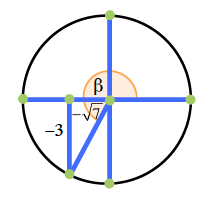### Home > PC3 > Chapter 11 > Lesson 11.1.3 > Problem11-51

11-51.

Given $\cot(β) =\frac { \sqrt { 7 } } { 3 }$ and $β$ is in Quadrant III $(π < β <\frac { 3 \pi } { 2 })$, calculate each of the following values.

1. $\cos(β)$

What must the signs be for
$\cot(\beta)=\frac{\text{adjacent}}{\text{opposite}}=\frac{-\sqrt{7}}{-3}$Calculate the length of the hypotenuse in the diagram. Use it to determine $\cos(β)$.
1. $\csc(β)$